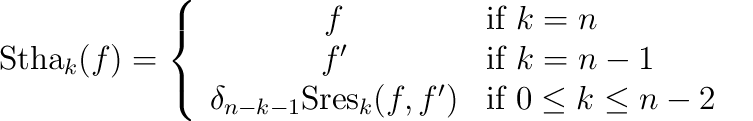## PolynomialTraits_d::SturmHabichtSequence

Note: This functor is optional!

### Definition

Computes the Sturm-Habicht sequence (aka the signed subresultant sequence) of a polynomial f of type PolynomialTraits_d::Polynomial_d with respect to a certain variable xi. The Sturm-Habicht sequence is similar to the polynomial subresultant sequence of f and its derivative f':=(∂f)/(∂xi) with respect to xi. The implementation is based on the following definition:

Let n:=deg f and δk:=(-1)k(k+1)/2. For k {0, ,n}, the k-th Sturm-Habicht polynomial of f is defined as:where Sresk(f,f') is defined as in the concept PolynomialTraits_d::PolynomialSubresultants.

The result is written in an output range, starting with the 0-th Sturm-Habicht polynomial (which is equal to the discriminant of f up to a multiple of the leading coefficient).

### Operations

 template OutputIterator fo ( Polynomial_d f , OutputIterator out ) computes the Sturm-Habicht sequence of f, with respect to the outermost variable. Each element is of type PolynomialTraits_d::Polynomial_d. template OutputIterator fo ( Polynomial_d f , OutputIterator out , int i ) computes the Sturm-Habicht sequence of f with respect to the variable xi.